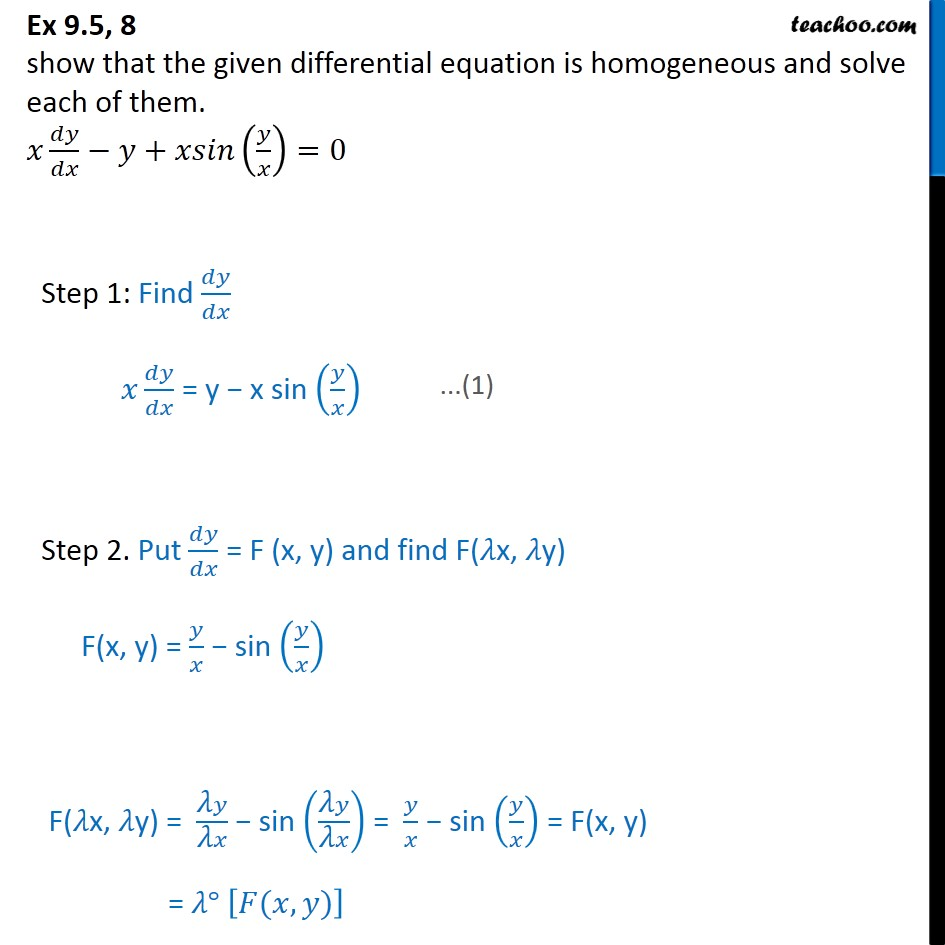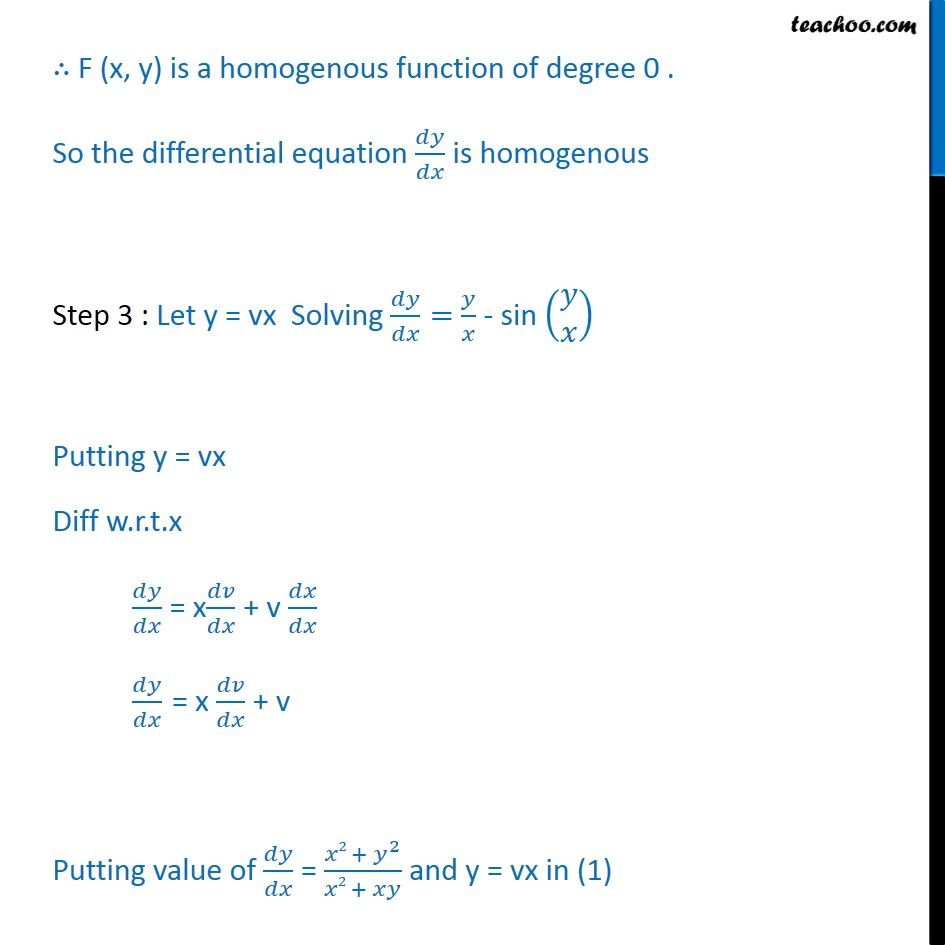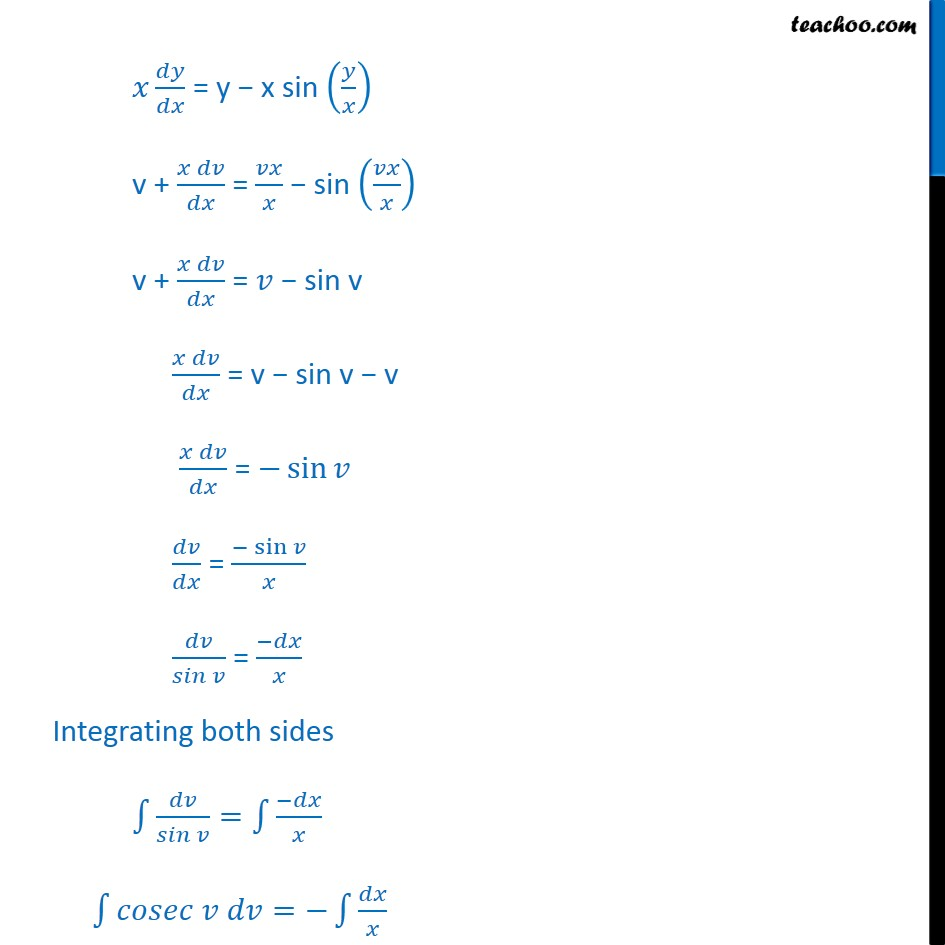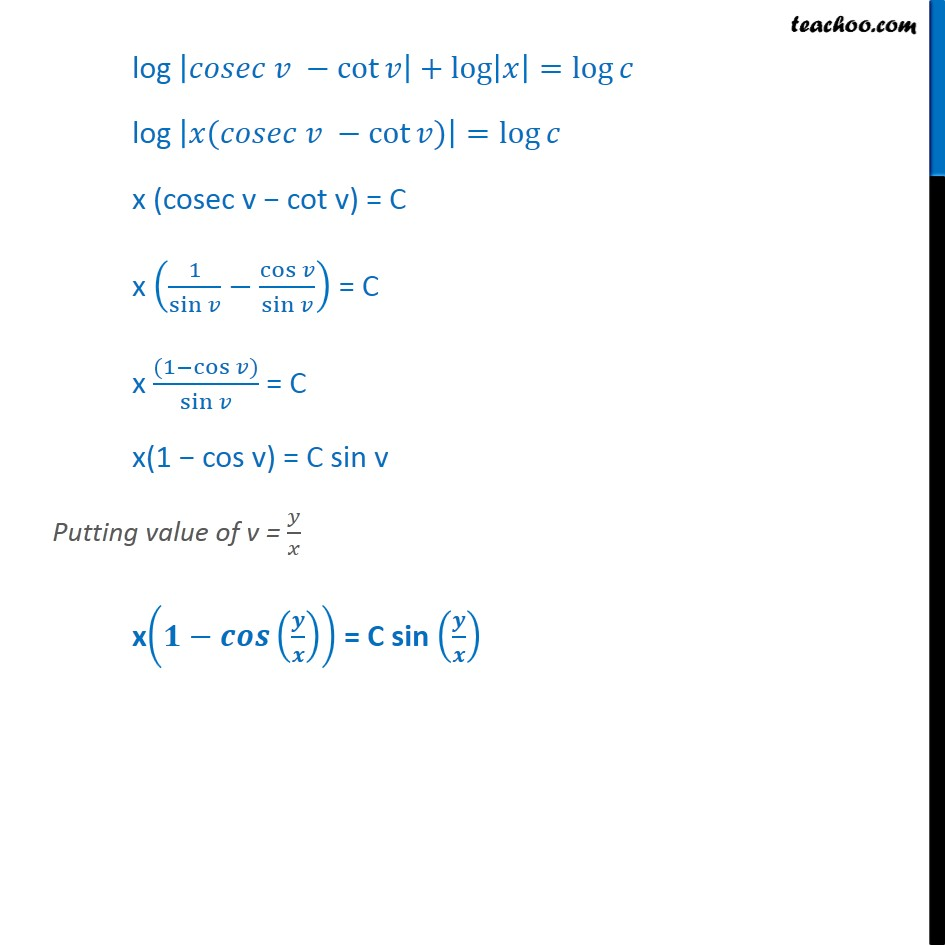1. Chapter 9 Class 12 Differential Equations
2. Serial order wise
3. Ex 9.5

Transcript

Ex 9.5, 8 show that the given differential equation is homogeneous and solve each of them. + =0 Step 1: Find = y x sin Step 2. Put = F (x, y) and find F( x, y) F(x, y) = sin F( x, y) = sin = sin = F(x, y) = ( , ) F (x, y) is a homogenous function of degree 0 . So the differential equation is homogenous Step 3 : Let y = vx Solving = - sin Putting y = vx Diff w.r.t.x = x + v = x + v Putting value of = 2 + 2 2 + and y = vx in (1) = y x sin v + = sin v + = sin v = v sin v v = sin = sin = Integrating both sides = = log cot = log + log log cot + log = log log ( cot ) = log x (cosec v cot v) = C x 1 sin cos sin = C x (1 cos ) sin = C x(1 cos v) = C sin v Putting value of v = x = C sin

Ex 9.5